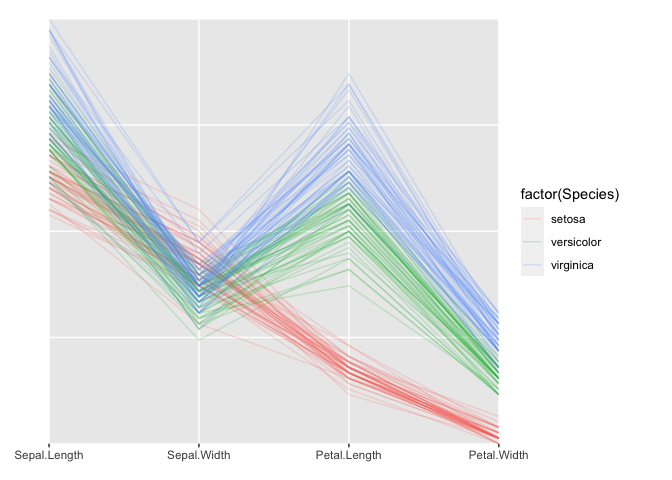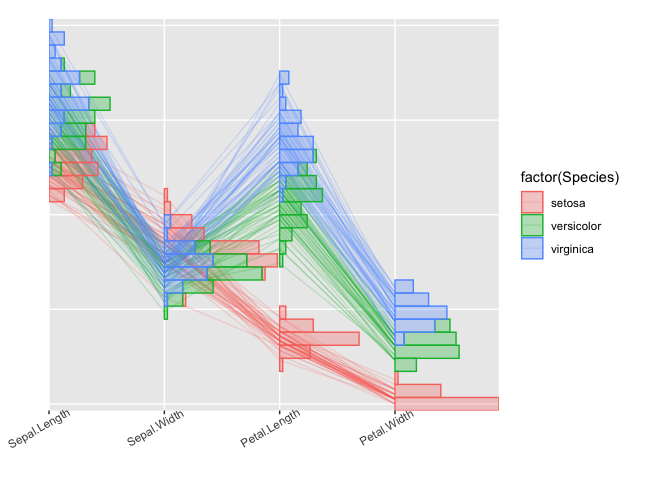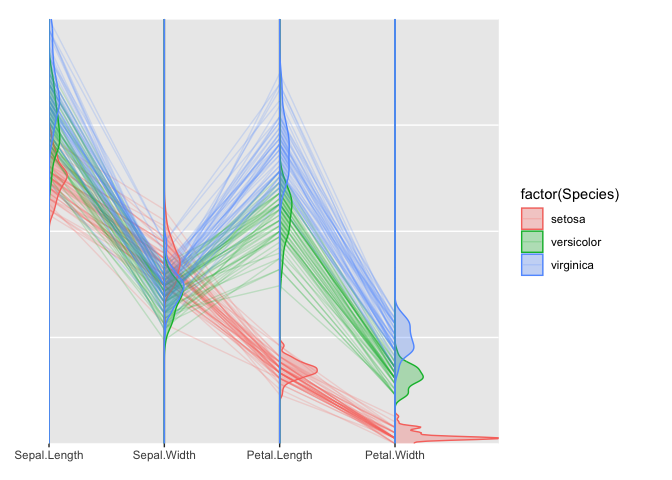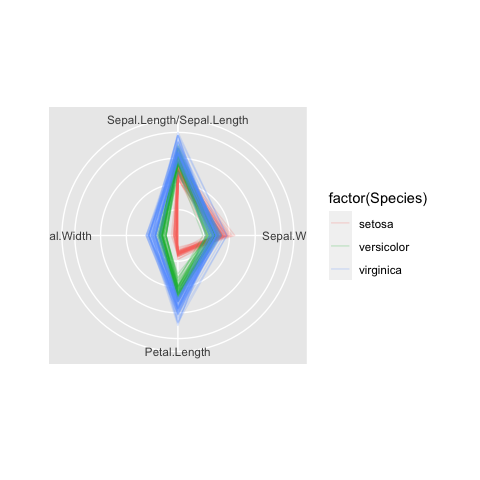# High Dimensional Data Visualization

## Serialaxes coordinate

Serial axes coordinate is a methodology for visualizing the $$p$$-dimensional geometry and multivariate data. As the name suggested, all axes are shown in serial. The axes can be a finite $$p$$ space or transformed to an infinite space (e.g. Fourier transformation).

In the finite $$p$$ space, all axes can be displayed in parallel which is known as the parallel coordinate; also, all axes can be displayed under a polar coordinate that is often known as the radial coordinate or radar plot. In the infinite space, a mathematical transformation is often applied. More details will be explained in the sub-section Infinite axes

A point in Euclidean $$p$$-space $$R^p$$ is represented as a polyline in serial axes coordinate, it is found that a point <–> line duality is induced in the Euclidean plane $$R^2$$ .

Before we start, a couple of things should be noticed:

• In the serial axes coordinate system, no x or y (even group) are required; but other aesthetics, such as colour, fill, size, etc, are accommodated.

• Layer geom_path is used to draw the serial lines; layer geom_histogram, geom_quantiles, and geom_density are used to draw the histograms, quantiles (not quantile regression) and densities. Users can also customize their own layer (i.e. geom_boxplot, geom_violin, etc) by editing function add_serialaxes_layers.

### Finite axes

Suppose we are interested in the data set iris. A parallel coordinate chart can be created as followings:

library(ggmulti)
# parallel axes plot
ggplot(iris,
mapping = aes(
Sepal.Length = Sepal.Length,
Sepal.Width = Sepal.Width,
Petal.Length = Petal.Length,
Petal.Width = Petal.Width,
colour = factor(Species))) +
geom_path(alpha = 0.2)  +
coord_serialaxes() -> p
pA histogram layer can be displayed by adding layer geom_histogram

p +
geom_histogram(alpha = 0.3,
mapping = aes(fill = factor(Species))) +
theme(axis.text.x = element_text(angle = 30, hjust = 0.7))A density layer can be drawn by adding layer geom_density

p +
geom_density(alpha = 0.3,
mapping = aes(fill = factor(Species)))A parallel coordinate can be converted to radial coordinate by setting axes.layout = "radial" in function coord_serialaxes.

p$coordinates$axes.layout <- "radial"
pNote that: layers, such as geom_histogram, geom_density, etc, are not implemented in the radial coordinate yet.

### Infinite axes

Andrews (1972) plot is a way to project multi-response observations into a function $$f(t)$$, by defining $$f(t)$$ as an inner product of the observed values of responses and orthonormal functions in $$t$$

$f_{y_i}(t) = <\mathbf{y}_i, \mathbf{a}_t>$

where $$\mathbf{y}_i$$ is the $$i$$th responses and $$\mathbf{a}_t$$ is the orthonormal functions under certain interval. Andrew suggests to use the Fourier transformation

$\mathbf{a}_t = \{\frac{1}{\sqrt{2}}, \sin(t), \cos(t), \sin(2t), \cos(2t), ...\}$

which are orthonormal on interval $$(-\pi, \pi)$$. In other word, we can project a $$p$$ dimensional space to an infinite $$(-\pi, \pi)$$ space. The following figure illustrates how to construct an “Andrew’s plot”.

p <- ggplot(iris,
mapping = aes(Sepal.Length = Sepal.Length,
Sepal.Width = Sepal.Width,
Petal.Length = Petal.Length,
Petal.Width = Petal.Width,
colour = Species)) +
geom_path(alpha = 0.2,
stat = "dotProduct")  +
coord_serialaxes()
p